# GSEB Solutions Class 12 Physics Chapter 14 Semiconductor Electronics: Materials, Devices and Simple Circuits

Gujarat Board GSEB Textbook Solutions Class 12 Physics Chapter 14 Semiconductor Electronics: Materials, Devices and Simple Circuits Textbook Questions and Answers, Additional Important Questions, Notes Pdf.

## Gujarat Board Textbook Solutions Class 12 Physics Chapter 14 Semiconductor Electronics: Materials, Devices and Simple Circuits

### GSEB Class 12 Physics Semiconductor Electronics: Materials, Devices and Simple Circuits Text Book Questions and AnswersQuestion 1.
In an n-type silicon, which of the following statement is true?
(a) Electrons are majority carriers and trivalent atoms are the dopants.
(b) Electrons arc minority carriers and pentavalent atoms are the dopants.
(c) Holes arc minority carriers and pentavalent atoms are the dopants.
(d) Holes are the majority carriers and trivalent atoms are the dopants.
(c) Holes arc minority carriers and pentavalent atoms are the dopants.

Question 2.
Which of the statements given in Exercise.1 is true for p-type semiconductors?
A p-type semiconductor is obtained by doping a semiconductor with a trivalent impurity. The impurity so added produces holes. Therefore, in a p-type semiconductor holes are the majority carries and trivalent atoms are the dopants. Hence, option (d) is correct.

Question 3.
Carbon, silicon, and germanium have four valence electrons each. These are characterized by valence and conduction bands separated by energy bandgap respectively equal to (Eg)C (Eg)Si and (Eg)Ge. Which of the following statements is true?
(a) (Eg)si < (Eg)Ge < (Eg)C
(b) (Eg)C < (Eg)Ge > (Eg)si
(c) (Eg)C > (Eg)si > (Eg)Ge
(d) (Eg)C = (Eg)si = (Eg)Ge
(c) (Eg)C > (Eg)si > (Eg)GeQuestion 4.
In an unbiased p-n junction, holes diffuse from the p-region to the n-region because
(a) free electrons in the n-region attract them.
(b) they move across the junction by the potential difference.
(c) hole concentration in p-region is more as compared to n-region.
(d) All the above
(c) hole concentration in p-region is more as compared to n-region.

Question 5.
When a forward bias is applied to a p-n junction, it
(a) raises the potential barrier.
(b) reduces the majority carrier current to zero.
(c) lowers the potential barrier.
(d) None of these
(c) lowers the potential barrier.

Question 6.
For transistor action, which of the following statements are correct?
(a) Base, emitter and collector regions should have similar size and doping concentrations.
(b) The base region must be very thin and lightly doped.
(c) The emitter junction is forward biased and the collector junction is reverse biased.
(d) Both the emitter junction as well as the collector junction are forward biased.
(b) The base region must be very thin and lightly doped.
and
(c) The emitter junction is forward biased and the collector junction is reverse biased.Question 7.
For a transistor amplifier, the voltage gain
(a) remains constant for all frequencies.
(b) is high at high and low frequencies and constant in the middle-frequency range.
(c) is low at high and low frequencies and constant at mid frequencies.
(d) None of these.
(c) is low at high and low frequencies and constant at mid frequencies.

Question 8.
In half-wave rectification, what is the output frequency if the input frequency is 50 Hz? What is the output frequency of a full-wave rectifier for the same input frequency?

• In a half-wave rectifier, the frequency of the output is the same as that of the input i.e. 50 Hz.
• In a full-wave rectifier, the frequency of the output is twice as that input i.e 100Hz.

Question 9.
For a CE-transistor amplifier, the audio signal voltage across the collected resistance of 2 kΩ is 2 V. Suppose the current amplification factor of the transistor is 100, find the input signal voltage and base current if the base resistance is 1 k Ω.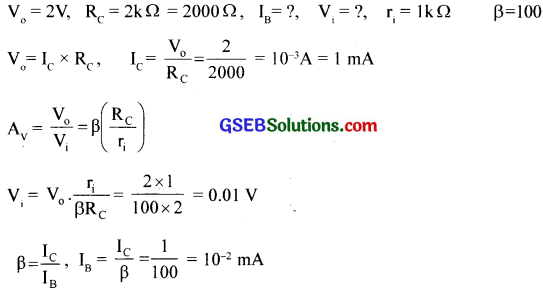Question 10.
Two amplifiers are connected one after the other in series (cascaded). The first amplifier has a voltage gain of 10 and the second has a voltage gain of 20. If the input signal is 0.01 volt, calculate the output ac signal.
A1 = 10, A2 = 20, Vi = 0.01 V, V0 = ?
Av = A1 x A2 = 10 x 20 = 200
Av = $$\frac{V_{0}}{V_{i}}$$, VB = Av x Vi = 200 x 0.01 = 2 VQuestion 11.
A p-n photodiode is fabricated from a semiconductor with a bandgap of 2.8 eY. Can it detect a wavelength of 6000 nm?
Eg = 2.8 eV, λ = 6000 nm = 6000 x 10-9m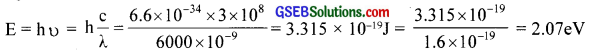No. Since ho is less than E8

Question 12.
In an intrinsic semiconductor, the energy gap Eg is 1.2eV. Its hole mobility is much smaller than electron mobility and independent of temperature. What are the ratio between conductivity at 600K and that at 300K? Assume that the temperature dependence of intrinsic carrier concentration ni is given by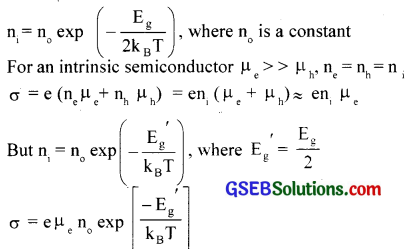Here all the pre-exponential terms are assumed to be independent of temperature. So we can put a constant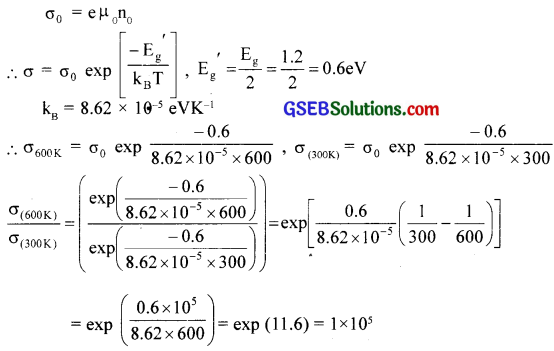This shows that the conductivity of a semiconductor increases rapidly with rise in temperature.Question 13.
You are given the two circuits as shown in fig. Show that circuit (a) acts as OR gate while circuit (b) acts as AND gate.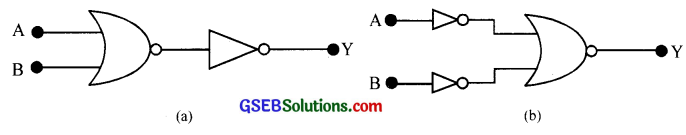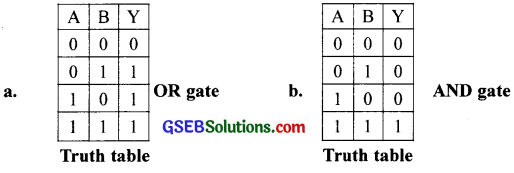Question 14.
Write the truth table for a NAND gate connected as given in the figure below.Hence identify the exact logic operation carried out by this circuit.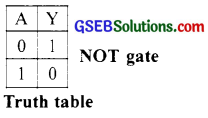Question 15.
You are given two circuits as shown in fig., which consist of NAND gates. Identify the logic operation carried out by the two circuits.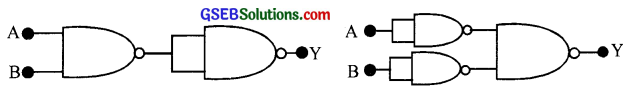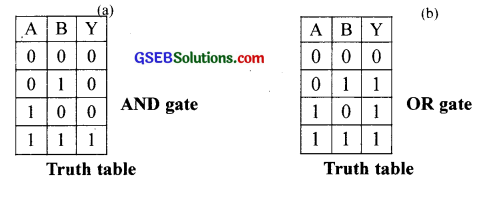Question 16.
Write the truth table for the circuit given in the figure below consisting of NOR gates and identify the logic operation (OR, AND, NOT) which this circuit is performing.(Hint: A = 0, B = 1, then A and B inputs of second NOR gate will be 0 and hence Y = 1. Similarly, work out the values of Y for other combinations of A and B. Compare with the truth table of OR, AND, NOT gates and find the correct one.)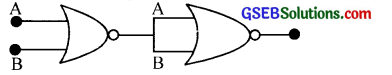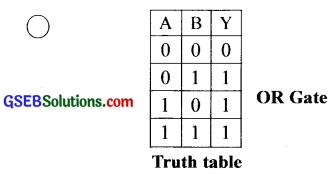Question 17.
Write the truth table for the circuits given in the figure consisting of NOR gates only. Identify the logic operations (OR, AND, NOT) performed by the two circuits.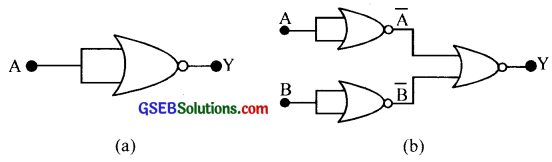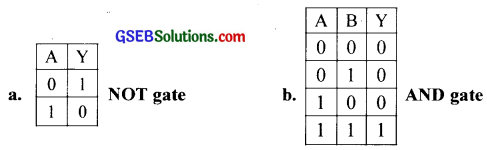### GSEB Class 12 Physics Semiconductor Electronics: Materials, Devices and Simple Circuits Additional Important Questions and Answers

Question 1.
The energy gap of diamond is 6 eV
(a) What does it mean:’
(b) What is (he value of energy gap in the case of copper?
(c) On the basis of energy gap. explain how substances are classified.
(a) In order to cross the energy barriers, an energy of 6 eV is required.
(b) zero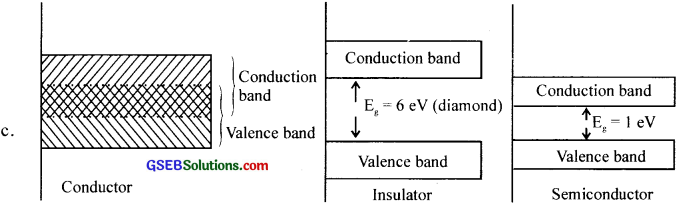Question 2.
When an impurity (atom) is added to an intrinsic semiconductor, then it becomes an extrinsic semiconductor.
(a) What is the above process called?
(b) What is the advantage of doing so?
(c) Distinguish between the intrinsic semiconductor and extrinsic semiconductor.
(a) Doping
(b) It increases the conductivity of semiconductors.
(c) Semiconductor (original) without impurity is called intrinsic and the one doped is called extrinsic semiconductors.

Question 3.
Fig shows a p-n junction diode.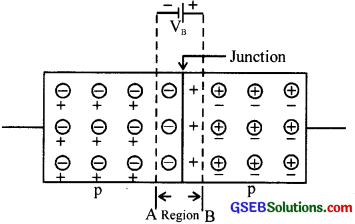(a) What does VB denote?
(b) Name the region AB.
(a) Potential barrier
(b) Depletion region

Question 4.
What is the value of conductivity of a semiconductor at absolute zero?
ZeroQuestion 5.
Can a transistor amplifier generate power?
No

Question 6.
Give some properties of a semiconductor

• It has covalent bonding
• It is crystalline in nature
• It has a negative temperature coefficient of resistance.
• Its conductivity increases with the addition of impurities.

Question 7.
What is a Zener diode?
A junction diode which can operate in the reverse break down voltage region is called Zener diode.

Question 8.
What are the characteristics of a hole?

• Holes carry a unit positive charge.
• Holes have the same charge as that of an electron.
• The energy of the hole is high as compared to that of the electron.
• The mobility of hole is smaller than that of electron.

Question 9.
Copy and complete the following block diagram.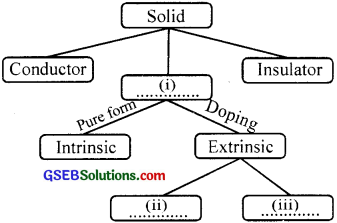1. Semiconductor
2. p-type
3. n-type

Question 10.
What is meant by forbidden energy gap?
The band separating the valence band and the conduction band is called forbidden gap.

Question 11.
What do you mean by Fermi energy?
The highest level occupied by the electrons at absolute zero is called Fermi level and the energy corresponding to this level is called Fermi energy.

Question 12.
What is the depletion region in the p-n junction?
A thin layer between p and n regions of the junction diode devoid of free electrons and holes is called depletion layer.Question 13.
What will happen both, emitter and collector of a transistor are reverse biased?
No conduction takes place.

Question 14.
How does the conductivity of a semi conductor increase?
By increasing the temperature or by doping with suitable impurities

Question 15.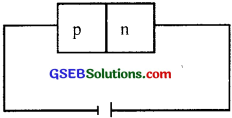The forward bias of a diode is wrongly given above.
(a) Redraw the above circuit correctly
(b) Draw the graph of current I with voltage V in forwarding bias
(c) Classify the following circuit diagram into forwarding bias, reverse bias, unbias.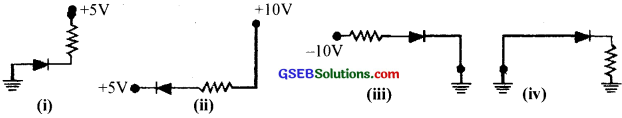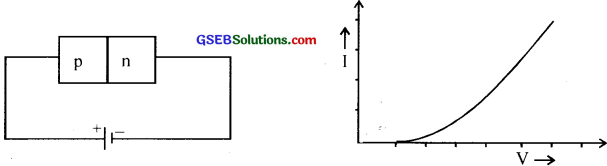(c) i. Reverse bias
ii. Forward bias
iii. Reverse bias iv. Unbias

Question 16.
Classify the following into conductors. insulators and semiconductors. Ga As, in P, Ni, Calcite, Graphite

• Conductors – Graphite. Ni
• Insulators – Calcite
• Semiconductors – GaAs, in P

Question 17.
The magnitude of potential harrier of germanium is about 0.3 e V
(a) What does it mean?
(b) What is the value of potential barrier for silicon?
(a) The depletion layer has the p.d = 0.3 eV. In order to pass current through the junction. a voltage > 0.3 V is required.
(b) 0.7V

Question 18.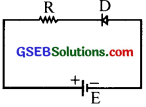(a) What type of biasing is used here?
(b) What happens to the depletion region?
(c) Will the diode conduct or not?
(a) Reverse biasing
(b) Becomes thicker
(c) NoQuestion 19.
What is a NOT gate known as un inverter?
A NOT gate always inverts the input. Hence a NOT gate is known as an inverter.

Question 20.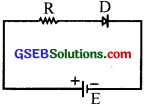(a) What type of biasing IC used here?
(b) What happens to the depletion region?
(c) Will the diode conduct or not?
(a) Forward biasing
(b) Becomes thinner
(c) Yes

Question 21.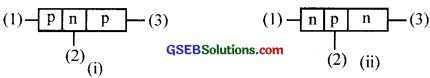The above figures represent a p-n-p transistor and an ti-p-n transistor.
(a) Draw the circuit symbols of p-n-p transistor and n-p-n transistor.
(b) Write the names of the terminals (1), (2) and (3,).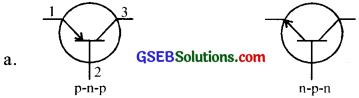(b) (1)Emitter
(2) Base
(3)Collector

Question 22.
In the normal working of a transistor, the emitter is forward biased and the collector is reverse biased.
(a) Comment on ¡lie above statement.
(b) can we exchange emitter and collector of a transistor?
(c) Base region of a transistor is made very thin and lightly doped. Why?
(d) “Even though the collector-base is reverse biased, the current ow takes place without experiencing much high resistance”. Give ‘our explanation.
(a) The movement of electrons from emitter to a collector in n-p-n and holes in p-n-p takes place only if the connections are made with emitter forward biased and collector reverse biased.

(b) No

(c) A thin and lightly dopped base region will contain a smaller number of majority charge carriers. This will reduce the recombination rate of electrons and holes when the majority charge carriers move from emitter to collector.

(d) The reverse potential value is higher than the forward-biased potential so that the charges injected to the base region are pulled effectively to the collector region.

Question 23.
A transistor is being used as a common emitter amplifier.
(a) What is the phase relationship between the output and input voltages?
(b) Define voltage gain of an amplifier
(c) Define the transconductance of a transistor.
(a) A phase change is introduced between input and output signal.

(b) Voltage gain, A of a transistor amplifier is the ratio of O/P voltage (Vo) to 1/P voltage (V1).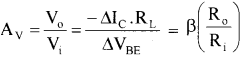(c) Transconductance, is the ratio of change in collector current to the change in the base-emitter voltage.
i.e., gm = $$\frac{\Delta \mathrm{I}_{\mathrm{C}}}{\Delta \mathrm{V}_{\mathrm{BE}}}$$
Also gm = $$\frac{\left|A_{V}\right|}{R_{L}}$$

Question 24.
Why does the conductivity of a semiconductor increase with rise of temperature?
When the temperature of a semiconductor increases, more and more electrons jump from the valence band to the conduction band (across the forbidden gap).Question 25.
Why are Ge and Si semiconductors?
The energy gaps in Ge and Si are of the order of 1 eV. Electrons can be easily excited from valence band to the conduction band to enable them to conduct electricity. So Ge and Si behave as semiconductors.

Question 26.
Why is a common emitter amplifier preferred over a common base amp amplifier?
The current gain of the common emitter amplifier is more than that of a common base amplifier.

Question 27.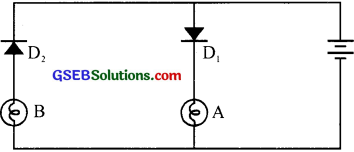(a) What do you observe when the circuit is closed?
(b) What will happen when the polarity of the cell is reserved? Why?
(a) Bulb A glows
(b) Bulb B glows because the diode D2 is forward biased

Question 28.
What are the different transistor configurations?

1. Common base configuration.
2. Common emitter configuration
3. Common collector configuration

Question 29.
Which configuration is widely used in circuits?
Common emitter. Because of high voltage and power gain.

Question 30.
What is the name of the device in which ac is converted into dc?
Rectifier

Question 31.
What is the name of the device in which dc is converted into ac?
OscillatorQuestion 32.
How can you keep the LC oscillation unhampered?
By providing a feedback circuit.

Question 33.
What the different gains in a transistor?
Current gain, voltage gain, power gain

Question 34.
What is a dark current?
Dark currents are reverse biased currents when no light falls on the photoed diode.

Question 35.
Transistor Radio does not work satisfactorily when used inside a railway carriage.
(b) What happens in a transistor when both the emitter and collector are reverse biased?
(c) What is this condition known as?
(d) Under what condition a transistor works as an open switch?
(a) The iron frame of the railway carriage acts as a magnetic screen. it does not allow the electromagnetic wave signal coming from transmitter to enter the carriage.
(b) No current flows through the transistor.
(c) Cutoff state
(d) When both the emitter and collector are reverse biased

Question 36.
Fill in the blanks with appropriate words given below
[Base, collector. emitter base-collector junction, collector-emitter junction. emitter haste junction]
Structurally a bipolar junction transistor consists of an emitter, base, and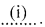Out of these regions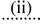is the most heavily doped. For proper functioning of a transistor.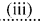is forward biased and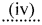is reverse biased.
i. collector
ii. emitter
iii. E-B Junction
iv. C-B Junction

Question 37.
A car stereo working at a stabilized voltage supply of 9V DC and has a Zener diode of 9V. 0.25 W Bia the voltage supply inside the car is 12 V DC.
The boy approached you to get help.
(a) Which mode of bias will you suggest to connect Zener diode us, voltage regulator?
(b) Draw a circuit diagram of voltage regulation to help the boy.
(c) Which device is essential for the circuit diagram? Find the value of that device.
[Hint: Current through the load, IL = 0]
(a) Reverse bias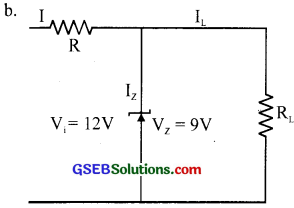(c) Resistance: R can be calculated using the equation Vi IR + Vz (R = 120 Ω)

Question 38.
State whether true or false and justify.
(a) Zener diodes are used under forwarding bias.
(b) In an n-p-n transistor, current conduction is primarily due to electrons.
(c) Transistor amplifier does not strictly obey the law of conservation of energy since the output power is greater than the input power.
(d) in a transistor amplifier, all the frequencies will have exactly equal gain.
(a) False. Zener diodes are used under reverse bias.
(b) True
(c) False
(d) FalseQuestion 39.
In both p and n-type semiconductors. actual/v electrons are flowing. What difference do you? observe in the motion of electrons in these two semiconductors?
Electrons in the valence band are flowing in a p-type semiconductor.
Electrons in the conduction band are flowing in the n-type semiconductor.

Question 40.
A greenhouse has an electronic system (block diagram is given below) which automatically switches ON a heater ¡f the air temperature in the greenhouse drops too low A manual switch is included so that the automatic system can be switched off
(Hint: The temperature sensor gives a logic – 1 output when the air temperature Ls normal and logic O when it is too cold)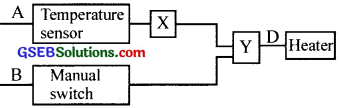(a) What is mean by I and O in the digital circuit?
(b) Name the logic gale Why is it used?
(c) Name the logic gate Y
(d) Construct a truth table of this electronic system by taking A and B as inputs and D as output.
(a) 1 – means maximum voltage
0 – means the minimum voltage

(b) NOT gate
To give I voltage at Y

(c) AND gate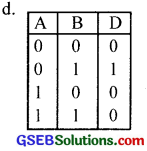Question 41.
The behaviour of the Control Unit of an automatic gas cooker is given below: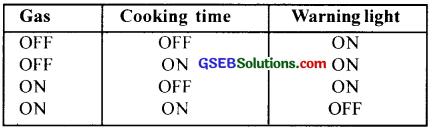Cooking time is different for different dishes. Hence cooking lime must be adjusted properly. This control unit can be constructed by using logic gates.
(a) What is meant by logic gates?
(b) Which gale is suitable for the above control unit?
(c) Construct a simple circuit diagram of the control unit
(Use symbol of the logic gate and block diagram of others)
(a) Electronic circuit in which the output follows some logical relationship with the input is called the logic gate.

(b) NAND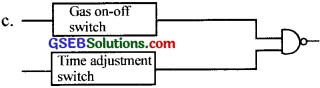Question 42.
Construct a truth table for the flowing logic circuit.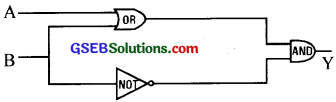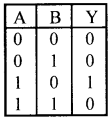Question 43.
Correct the following CE amplifier circuit.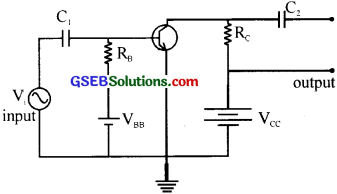Question 44.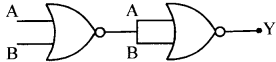(a) Name the gates in the combination.
(b) Identify the logic operation of the whole gate.
(c) Give the truth table.
(a) Double input NOR gate and single input NOR gate.
(b) $$\overline{A+B}$$ = A + B, an OR gate.

(c)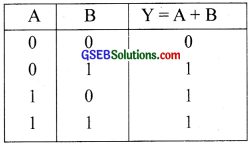Question 45.
Crystalline substances have a sharp melting point. Why?